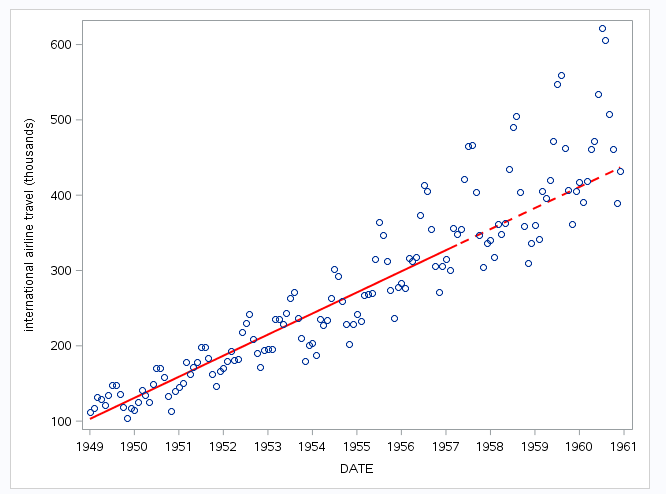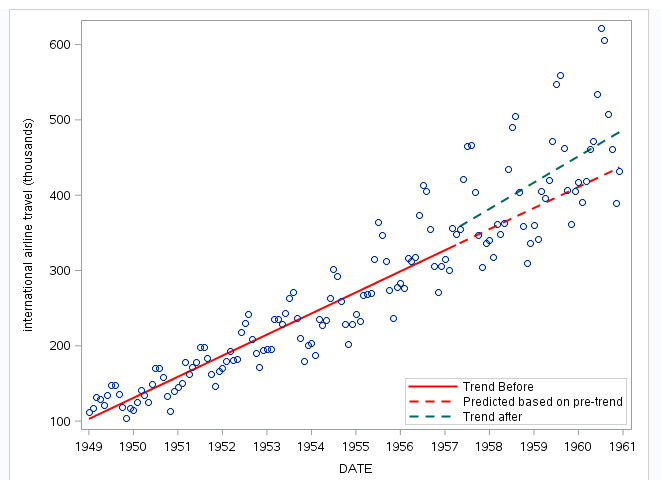## Time Series forecast plot

Hello,

I have data made from two variables: adm_date and total_nr (example below):

1 200

2 265

3 245

...

69 340

70 356

I want to do two things:

1. I want to use first 59 observations and forecast the rest.

2. I want to plot regression line and real data in same graph, similar to example below (I do not need that grey block):8 REPLIES 8Ksharp
Super User

## Re: Time Series forecast plot

``````%let n=100;
data have;
set sashelp.air;
retain group 1;
if _n_ gt &n then do;group=2;air=.;end;
run;

proc reg data=have outest=est(keep=date rename=(date=slope)) noprint;
model air=date;
output out=pred predicted=pred;
quit;

data want;
merge sashelp.air pred(keep=date pred group rename=(date=_date));
run;

ods graphics / attrpriority=none;
proc sgplot data=want noautolegend;
styleattrs datalinepatterns=(solid dash);
series x=_date y=pred/group=group lineattrs=(color=red thickness=2);
scatter x=date y=air;
run;``````## Re: Time Series forecast plot

Thanks!

And maybe you know how i could add another regression line only for the time period I forecasted, but this time to use the real data points?

## Re: Time Series forecast plot

Is it some other regression line, or you simply do not want to see the historical region in your plot? If it is the latter, the default forecast that is produced already does that. If it is another regression line, you will have to use the SGPLOT procedure with the UCM output data set (that is the simplest way I can think of). Use the LINEPARM, SERIES and SCATTER statements in PROC SGPLOT.

## Re: Time Series forecast plot

There are many was to do this (one already shown).  This is yet another (see the back= and lead= options in the ESTIMATE and FORECAST statements):

proc ucm data=sashelp.air;

id date interval=month; *optional;

model air;

irregular;

level variance=0 noest;

slope variance=0 noest;

estimate back=20; *sasy;

run;

This will produce a plot that is based on first 124 observations (out of 144).  The plot has confidence bands around them.  If you don't want them, you can use sgplot  with the columns in the output data set, for, (s_level has the values of the fitted line).  See the UCM doc for more info: http://support.sas.com/documentation/cdl/en/etsug/68148/HTML/default/viewer.htm#etsug_ucm_toc.htm

## Re: Time Series forecast plot

I am sorry if i am not clear, I would like to get a graph similar to (I want to see grapphically if there is any change due to policy implementation at specific time):Ksharp
Super User

## Re: Time Series forecast plot

``````%let n=100;
data have;
set sashelp.air;
retain group 1;
if _n_ gt &n then do;group=2;air=.;end;
run;
data have2;
set sashelp.air;
if _n_ gt &n ;
run;

proc reg data=have noprint;
model air=date;
output out=pred predicted=pred;
quit;
proc reg data=have2 noprint;
model air=date;
output out=pred2 predicted=pred;
quit;

data want;
merge sashelp.air pred(keep=date pred group rename=(date=_date))
pred2(keep=date pred rename=(date=_date2 pred=pred2));
run;

ods graphics / attrpriority=none;
proc sgplot data=want noautolegend;
styleattrs datalinepatterns=(solid dash);
series x=_date y=pred/group=group lineattrs=(color=red thickness=2);
series x=_date2 y=pred2/lineattrs=graphdata3(thickness=2 pattern=dash);
scatter x=date y=air;
run;``````

## Re: Time Series forecast plot

Thanks!

What is the best way in this case to put legend in graph?Ksharp
Super User

## Re: Time Series forecast plot

``````%let n=100;
data have;
set sashelp.air;
retain group 'Trend Before                            ';
if _n_ gt &n then do;group='Predicted based on pre-trend';air=.;end;
run;
data have2;
set sashelp.air;
if _n_ gt &n ;
run;

proc reg data=have noprint;
model air=date;
output out=pred predicted=pred;
quit;
proc reg data=have2 noprint;
model air=date;
output out=pred2 predicted=pred;
quit;

data want;
merge sashelp.air pred(keep=date pred group rename=(date=_date))
pred2(keep=date pred rename=(date=_date2 pred=pred2));
run;

ods graphics / attrpriority=none;
proc sgplot data=want ;
styleattrs datalinepatterns=(solid dash);
series x=_date y=pred/group=group lineattrs=(color=red thickness=2)
name='a' legendlabel=' ';
series x=_date2 y=pred2/lineattrs=graphdata3(thickness=2 pattern=dash)
name='b' legendlabel='Trend after';
scatter x=date y=air;
keylegend 'a' 'b'/ location=inside position=bottomright across=1;
run;``````Discussion stats
• 8 replies
• 1034 views
• 0 likes
• 3 in conversation